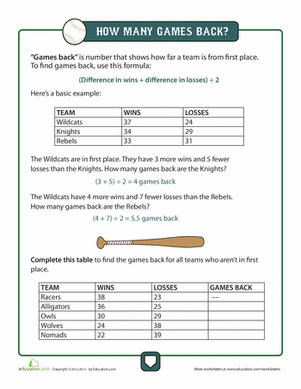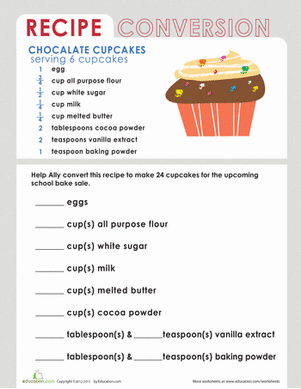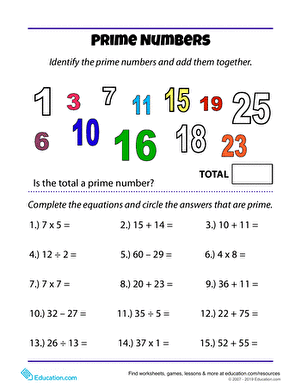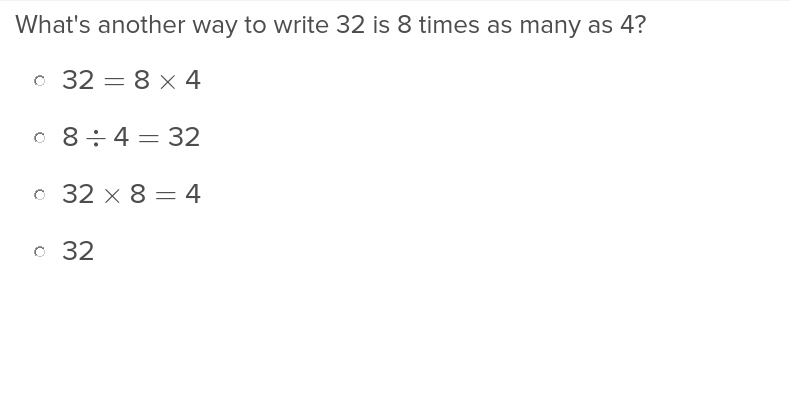Learning Library
premium

# 4.OA.A Worksheets, Workbooks, Lesson Plans, and Games

#### CCSS.MATH.CONTENT.4.OA.A

:
"Use the four operations with whole numbers to solve problems."

These worksheets and exercises can help students practice this Common Core State Standards skill.

## WorksheetsBaseball Math: Games Back
Worksheet
Baseball Math: Games Back
Baseball provides great opportunities to learn math concepts. Here's a concept, games back, that requires an algebra formula simple even for 4th grade.
4th grade
Math
WorksheetDry Measure Conversion
Worksheet
Dry Measure Conversion
Give recipe conversion practice a try by multiplying the ingredients in this cupcake recipe. Liquid and dry measure conversion are included; cups,…
4th grade
Math
WorksheetFinding Prime Numbers
Worksheet
Finding Prime Numbers
What is a prime number? A number is prime when it is only divisible by 1 and itself. Help your little mathematician recognize prime numbers.
4th grade
Math
Worksheet

## Lesson Plans

No plans found for this common core node.

## Workbooks

No workbooks found for this common core node.

## Games

No games found for this common core node.

## ExercisesPlace Value and Multiplicative Comparisons
Exercise
Place Value and Multiplicative Comparisons
Translate word problems into their numeric equivalent with this exercise that teaches both place value and multiplicative comparisons.
4th grade
Math
Exercise

### Add to collection

Create new collection

0

### New Collection>

0 items

What could we do to improve Education.com?

Please note: Use the Contact Us link at the bottom of our website for account-specific questions or issues.

What would make you love Education.com?